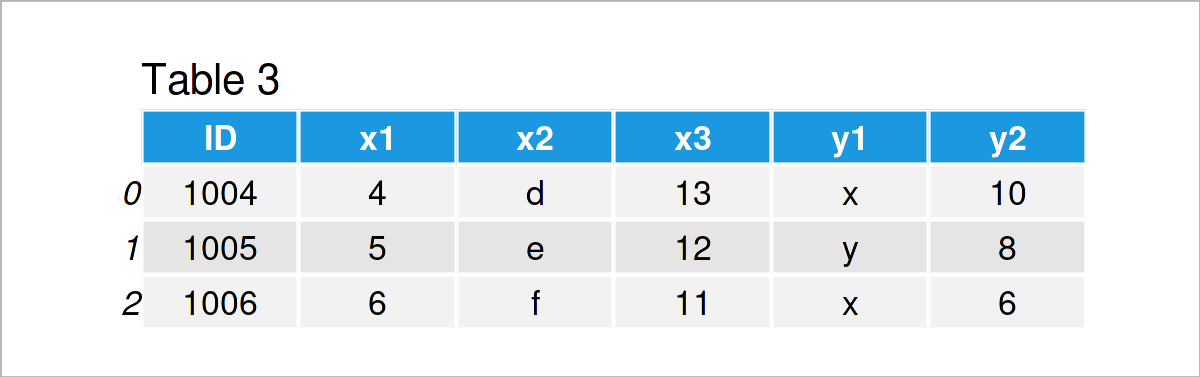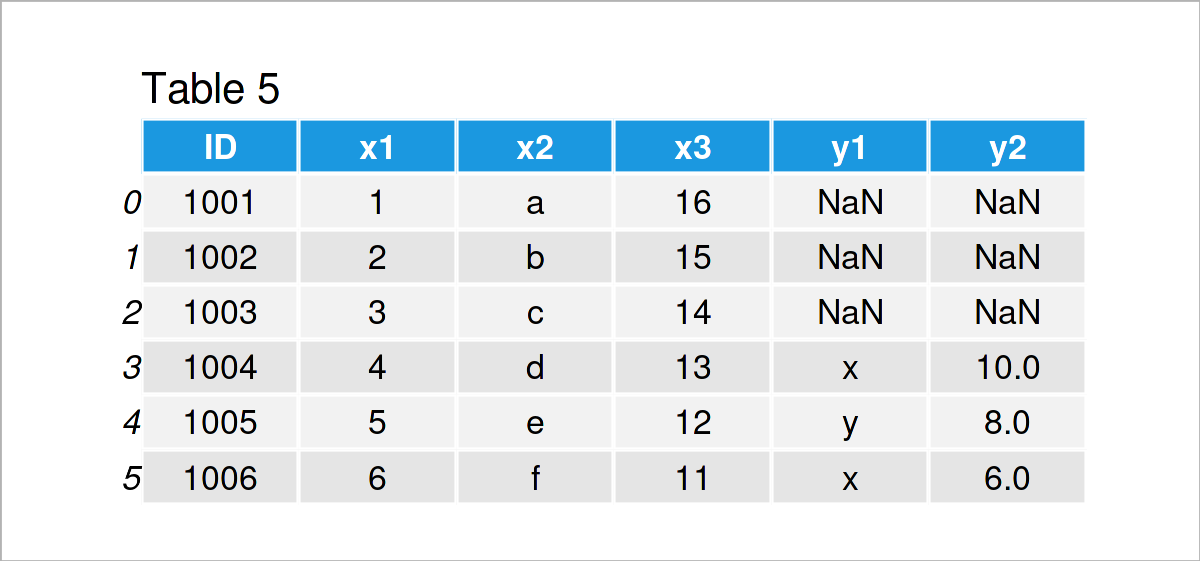# Types of Joins for pandas DataFrames in Python (4 Examples)

In this tutorial, I’ll demonstrate how to apply different types of joins in Python.

It’s time to dive into the exemplifying Python syntax…

## Overview of Different Types of Joins

It is important to note that Python DataFrames can be merged based on many different types of SQL joins.

The figure below illustrates four of the most popular kinds of joins:At the top of the figure, two different separate data sets are shown. At the bottom of the figure, you can see four different joined versions of these two data sets:

• Inner join: Keep only IDs that are contained in both data sets.
• Outer join: Keep all IDs.
• Left join: Keep only IDs that are contained in the first data set.
• Right join: Keep only IDs that are contained in the second data set.

All of these join-types will be applied in the following programming examples.

## Example Data & Add-On Libraries

First, we have to load the pandas library:

`import pandas as pd                              # Import pandas`

In addition, consider the following example data:

```data1 = pd.DataFrame({"ID":range(1001, 1007),    # Create first pandas DataFrame
"x1":range(1, 7),
"x2":["a", "b", "c", "d", "e", "f"],
"x3":range(16, 10, - 1)})
print(data1)                                     # Print first pandas DataFrame``````data2 = pd.DataFrame({"ID":range(1004, 1009),    # Create second pandas DataFrame
"y1":["x", "y", "x", "y", "x"],
"y2":range(10, 1, - 2)})
print(data2)                                     # Print second pandas DataFrame```After executing the previous Python syntax the two pandas DataFrames shown in Tables 1 and 2 have been created.

Note that both of these DataFrames contain an ID column. We will use this column to match the observations in our data sets.

## Example 1: Merge Two pandas DataFrames Using Inner Join

In this example, I’ll explain how to use an inner join to merge two pandas DataFrames.

For this task, we can use the merge function of the pandas package as shown below:

```data_inner = pd.merge(data1,                     # Inner join
data2,
on = "ID",
how = "inner")
print(data_inner)                                # Print merged DataFrame```The output of the previously shown Python code is shown in Table 3: We have created a combined union of our two input data sets.

Note that several rows of the input DataFrames have been excluded, because the corresponding IDs of these rows were only contained in one of the two data sets.

## Example 2: Merge Two pandas DataFrames Using Outer Join

In this example, I’ll demonstrate how to apply a full outer join in Python.

To do this, we have to specify the how argument within the merge function to be equal to “outer”:

```data_outer = pd.merge(data1,                     # Outer join
data2,
on = "ID",
how = "outer")
print(data_outer)                                # Print merged DataFrame```As shown in Table 4, we have created another merged pandas DataFrame.

As you can see, the outer join has kept all data that were stored in the two input data sets. In case an ID was only contained in one of the two DataFrames, NaN values have been inserted for the variables in the other DataFrame.

## Example 3: Merge Two pandas DataFrames Using Left Join

This example shows how to use a left join to combine two pandas DataFrames.

For this, we have to specify the how argument to be equal to “left”:

```data_left = pd.merge(data1,                      # Left join
data2,
on = "ID",
how = "left")
print(data_left)                                 # Print merged DataFrame```After running the previous Python code the pandas DataFrame shown in Table 5 has been created. As you can see, we have appended the columns of the second data set on the right side of the first data set.

All IDs of the left data set have been kept, but IDs only existing in the second data set have been removed.

## Example 4: Merge Two pandas DataFrames Using Right Join

This section illustrates the opposite join compared to Example 3, i.e. a right join.

We have to set the how argument to be equal to “right” to conduct this type of join:

```data_right = pd.merge(data1,                     # Right join
data2,
on = "ID",
how = "right")
print(data_right)                                # Print merged DataFrame```In Table 6 you can see that we have created another concatenated version of our input DataFrames using the previous syntax. This time, we have kept all IDs of the second pandas DataFrame

## Video & Further Resources

Note that we have only discussed the four most common kinds of joins in this tutorial. In case you want to learn more about less popular join algorithms such as self joins, semi joins, and anti joins, please have a look here and here.

This article has illustrated how to apply different types of joins for pandas DataFrames in Python. If you have additional questions, don’t hesitate to let me know in the comments.

Subscribe to the Statistics Globe Newsletter

•Salwa Mousa
June 9, 2022 1:33 pm

Dear sir
Can you send me in email the lecture of python

•June 10, 2022 7:09 am